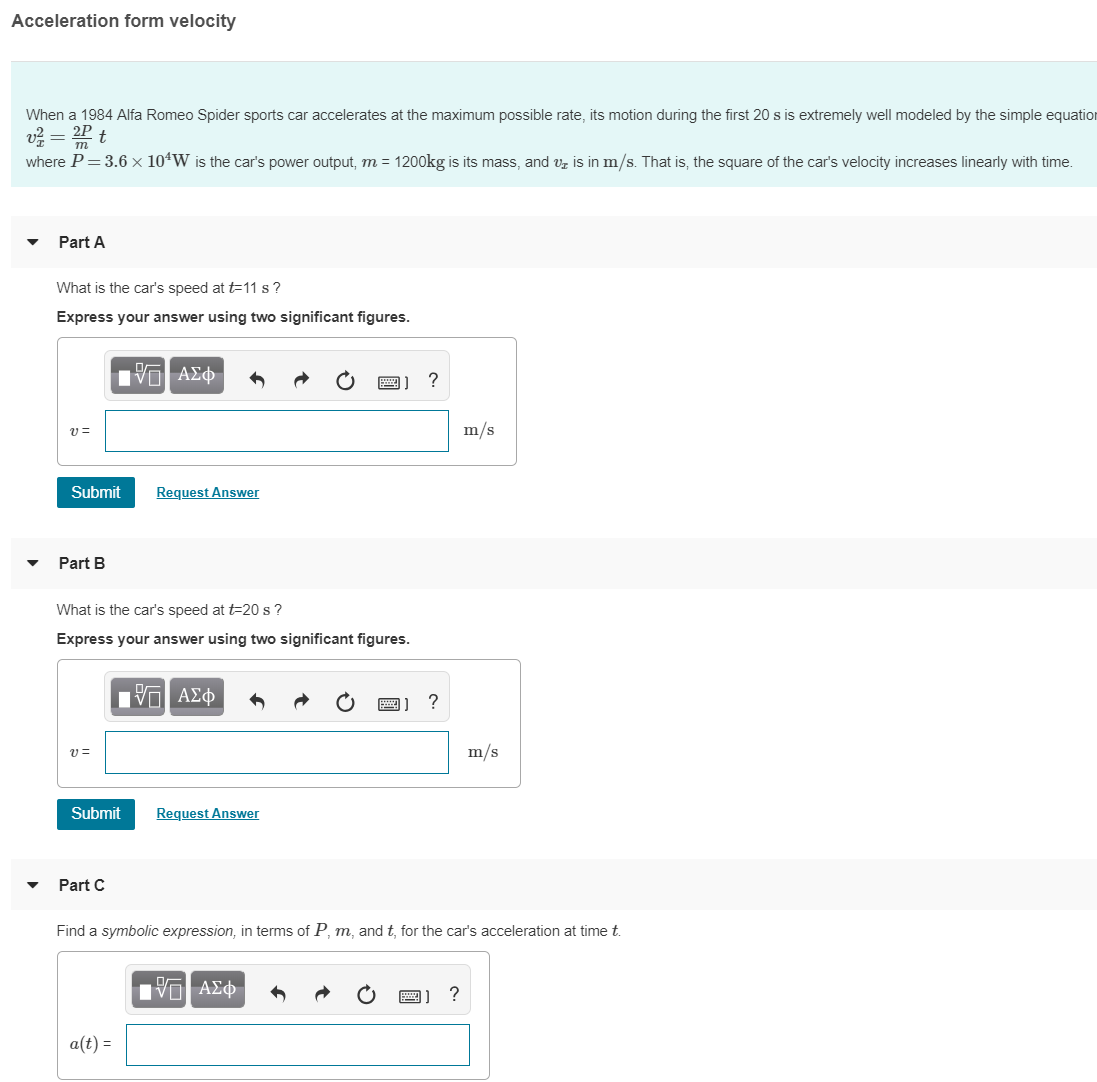# Acceleration form velocityWhen a 1984 Alfa Romeo Spider sports car accelerates at the maximum possible rate, its motion during the first 20 s is extremely well modeled by the simple equation222Pwhere P 3.6 x 10W is the car's power output, m 1200kg is its mass, and v is in m/s. That is, the square of the car's velocity increases linearly with timePart AWhat is the car's speed at t-11 s?Express your answer using two significant figuresu Em/sSubmitRequest AnswerPart BWhat is the car's speed at t-20 s?Express your answer using two significant figuresu Em/sSubmitRequest AnswerPart CFind a symbolic expression, in terms of P, m, and t, for the car's acceleration at time ta(t)-

Question
50 views

Need help with a, b, and c. Thank you!help_outlineImage TranscriptioncloseAcceleration form velocity When a 1984 Alfa Romeo Spider sports car accelerates at the maximum possible rate, its motion during the first 20 s is extremely well modeled by the simple equation 222P where P 3.6 x 10W is the car's power output, m 1200kg is its mass, and v is in m/s. That is, the square of the car's velocity increases linearly with time Part A What is the car's speed at t-11 s? Express your answer using two significant figures u E m/s Submit Request Answer Part B What is the car's speed at t-20 s? Express your answer using two significant figures u E m/s Submit Request Answer Part C Find a symbolic expression, in terms of P, m, and t, for the car's acceleration at time t a(t)- fullscreen
check_circle

Step 1

Given:

Step 2

Part (A):

The speed of car at t=11 s is given by,

Step 3

The value of speed in two signif...

### Want to see the full answer?

See Solution

#### Want to see this answer and more?

Solutions are written by subject experts who are available 24/7. Questions are typically answered within 1 hour.*

See Solution
*Response times may vary by subject and question.
Tagged in

### Physics